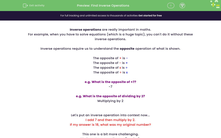# Find Inverse Operations

In this worksheet, students will find inverse operations and use these to work backwards from outcomes to starting points.Key stage:  KS 4

Year:  GCSE

GCSE Subjects:   Maths

GCSE Boards:   Pearson Edexcel, OCR, Eduqas, AQA,

Curriculum topic:   Number, Number Operations and Integers

Curriculum subtopic:   Structure and Calculation Inverse Operations

Difficulty level:#### Worksheet Overview

Inverse operations are really important in maths.

For example, when you have to solve equations (which is a huge topic), you can't do it without these inverse operations.

Inverse operations require us to understand the opposite operation of what is shown.

The opposite of + is -

The opposite of - is +

The opposite of x is ÷

The opposite of ÷ is x

e.g. What is the opposite of +7?

-7

e.g. What is the opposite of dividing by 2?

Multiplying by 2

Let's put an inverse operation into context now...

I add 7 and then multiply by 2.

If my answer is 18, what was my original number?

This one is a bit more challenging.

We have two operations: + 7 and x 2.

The inverse operations for these would be - 7 and ÷ 2.

But which operation do we use first?

If we are doing the opposite, we are moving backwards through the sum.

In the question, we added 7 first then multiplied by 2.

When we are doing the inverse, we swap this order as well.

So to solve this we divide by 2 first, then subtract 7:

18 ÷ 2 = 9

9 - 7 = 2

The original starting number was 2.

Now it's your turn to have a go!

In this activity, you will find inverse operations and use these to work backwards from outcomes to starting points.

### What is EdPlace?

We're your National Curriculum aligned online education content provider helping each child succeed in English, maths and science from year 1 to GCSE. With an EdPlace account you’ll be able to track and measure progress, helping each child achieve their best. We build confidence and attainment by personalising each child’s learning at a level that suits them.

Get started